Paul Murrell
The University of Auckland
July 2018

## Minard's Map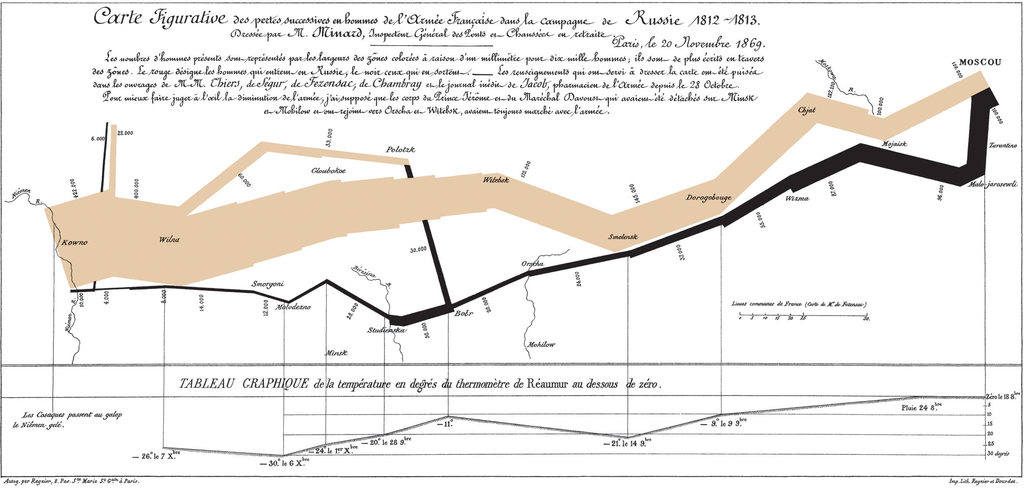This is Minard's famous map of Napolean's march on Moscow (and back). The important feature for this talk is the thick band that represents the size of Napoleon's army. The map was drawn in the 1840s(?) so did not benefit from software support. Thing is, it is not clear that modern software can help with drawing that thick band !

## Line Primitives

grid.segments(.2, .2, .8, .8, gp=gpar(lwd=20))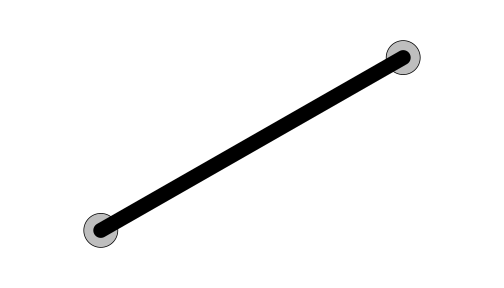Modern graphics systems typically have a set of line drawing primitives consisting of line segments ...

## Line Primitives

grid.lines(c(.2, .5, .8), c(.2, .8, .2),
gp=gpar(lwd=20))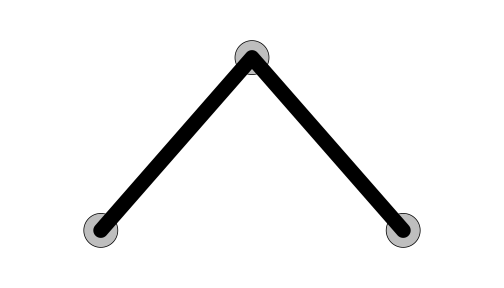... polylines ...

## Line Primitives

grid.xspline(c(.2, .5, .8), c(.2, .8, .2), shape=1,
gp=gpar(lwd=20))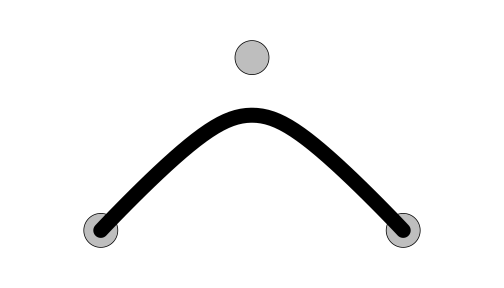... and smooth curves. This is an X-spline, which is a flexible curve primitive that draws a line relative to control points with a shape parameter that controls whether the line approximates a control point ...

## Line Primitives

grid.xspline(c(.2, .5, .8), c(.2, .8, .2), shape=-1,
gp=gpar(lwd=20))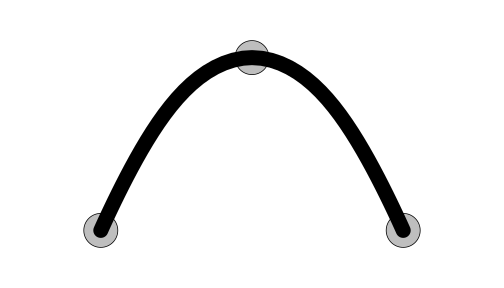... or interpolates a control point ...

## Line Primitives

grid.xspline(c(.2, .5, .8), c(.2, .8, .2), shape=0,
gp=gpar(lwd=20))... or is discontinuous at a control point.

## Line Primitives

grid.lines(c(.2, .5, .8), c(.2, .8, .2),
gp=gpar(lwd=20, lineend="butt", linejoin="mitre"))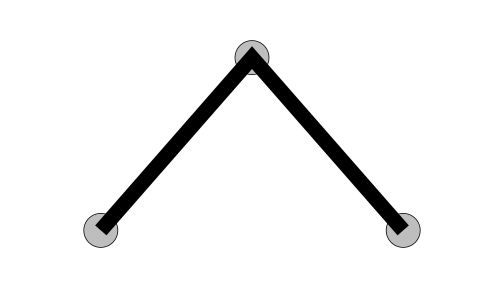Graphics systems also provide control over the style of line endings (round, square, butt) and line joins (round, mitre, bevel).

## Variable-Width LinesThe difficulty with Minard's map is that the thick band is a line with varying width.

## Variable-Width Lines

grid.segments(c(.2, .5), c(.2, .8), c(.5, .8), c(.8, .2),
gp=gpar(lwd=c(40, 80), lineend="butt"))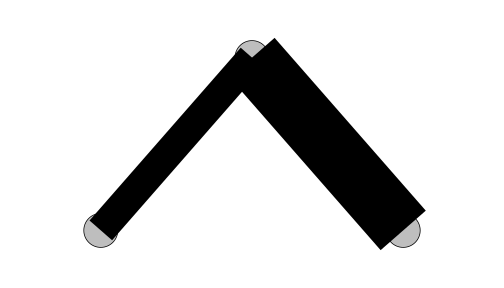Note that it is NOT just a series of line segments with different widths because the line joins matter.

## Variable-Width Lines

library(vwline)
grid.vwline(c(.2, .5, .8), c(.2, .8, .2),
w=c(.1, .2, .2),
stepWidth=TRUE, linejoin="mitre")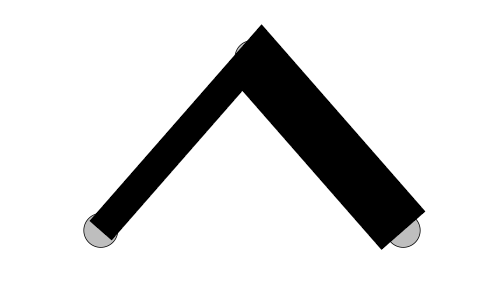All of which is an excuse to wonder: wouldn't it be fun to have a variable-width line primitive ? Hence the 'vwline' package.

## Variable-Width Lines

grid.vwcurve(x, y, w, ...)
grid.vwXspline(x, y, w, ...)
grid.vwline(x, y, w, ...)
grid.brushXspline(brush, x, y, w, ...)
grid.offsetXspline(x, y, w, ...)


There are many different approaches to generating a variable-width line. The 'vwline' package provides several functions for drawing a variable-width line relative to (x, y) locations or control points.

## Variable-Width LinesIn all cases, an algorithm is used to generate the outline of a variable-width line (a polygon or path) and then that outline is filled in.

## Variable-Width Lines

grid.vwcurve(x, y, w, ...)grid.vwcurve() is based on a series of points. We calculate a "perpendicular" at each point and then connect up the ends of the perpendiculars.

## Variable-Width Lines

grid.vwcurve(x, y, w, ...)The example on the left is based on just four points and the width changes smoothly between points, but the line itself has sharp corners. The example on the right is based on lots of points so the line appears smooth and the widths change smoothly.

## Variable-Width Lines

grid.vwXspline(x, y, w, ...)grid.vwXspline() is based on a set of control points. We generate new control points based on perpendiculars at the original control points, then connect up the curves through the new control points.

## Variable-Width Lines

grid.vwXspline(x, y, w, ...)These curves are always smooth, though the result is less predictable. The image on the left is based on just five control points. The image on the right is one main line with lots of smaller lines drawn outward from its extremities (in addition to drawing variable-width lines, we can query them for their boundary points)

## Variable-Width Lines

grid.vwline(x, y, w, ...)grid.vwline() is based on a set of points. We generate a thick line for each segment and then calculate a "join" for the outside and inside of each corner.

## Variable-Width Lines

grid.vwline(x, y, w, ...)Both of these lines are based on the same set of points - we have just changed the line ending and line join styles. The left image shows examples of "round" ends and joins, though these are not as simple as circle segments (as is the case for fixed-width lines). The right image shows an example of "stepped" changes in line width (with "square" line ends and "mitre" line joins).

## Variable-Width Lines

grid.brushXspline(brush, x, y, w, ...)grid.brushXspline() is based on a smooth line relative to a set of control points. A brush is placed at points along the smooth line (perpendicular to the line), then each "segment" is created from a convex hull around consecutuive brushes. The end result is a union of all of the segments.

## Variable-Width Lines

grid.brushXspline(brush, x, y, w, ...)The left image shows that we can place the brushes at relatively wide intervals to create a "broken" variable-width line. The right image is based on a circular X-spline with a half-brush that only draws "inside" the circle (at variable widths).

## Variable-Width Lines

grid.offsetXspline(x, y, w, ...)grid.offsetXspline() is based on a smooth line relative to control points. The smooth line is a series of very short straight lines. The offset curve for the original X-spline is used to calculate the width at each vertex on the "smooth" line. The width of the line is specified as an X-spline (where 'x' is distance along the line and 'y' is the width).

## Variable-Width Lines

grid.offsetXspline(x, y, w, ...)The left image shows that this mathematical (rather than heuristic) approach is better at coping with tight corners with rapidly changing width. The right image shows a width X-spline that smoothly decreases to zero then back up again, and repeats.

## An Application(!)

x <- matrix(c(1:10), 2,5)
par(lwd = 5)
barplot(x, beside=T, border=rep(c(NA, 'black'), 5),
space=c(0.08, 1), col=rep(c('black', 'white'), 5))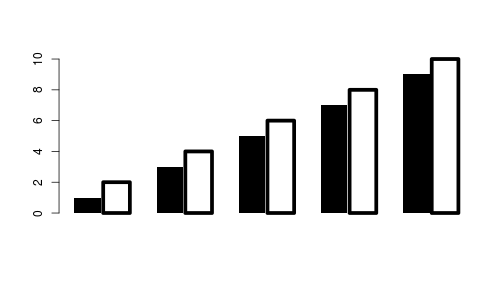Less than a year after doing this work, someone came up with a problem (on R-help) that makes use of this solution! The problem here is that the filled bars are only filled (so that the bottoms of the bars align with zero on the y-axis), but the unfilled bars are stroked with thick lines, which "bleeds" below zero and makes the bars look uneven. What we want is to have the borders on the unfilled bars only visible "inside" the bars.

## An Application(!)

grid.vwline(unit.c(left, left, right, right),
unit.c(bottom, top, top, bottom),
w=widthSpec(list(left=rep(0, 4),
right=unit(rep(1, 4), "mm"))),
open=FALSE)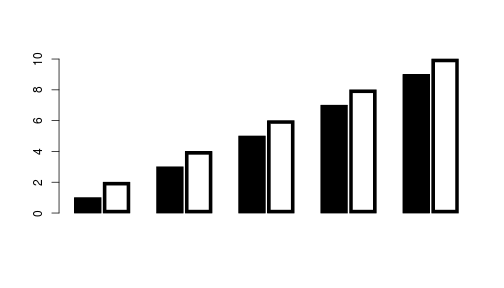Variable-width lines are one way to achieve this, by drawing a line (clockwise) around the border of the bar with a zero width on the left and a thick width on the right.

## Variable-Width Lines

grid.vwline(long, lat, default.units="native",
w=unit(survivors/maxSurvivors, "in"),
stepWidth=TRUE, linejoin="mitre")The grid.vwline() function, with stepWidth=TRUE and linejoin="mitre" can produce a variable-width line that changes width in abrupt steps. This gets us quite close to what Minard did, though I have to admit it is still not quite there. Notice though that the "return" line has variable-width on only one side! We can do that!

## Summary

The 'vwline' package for R draws variable-width lines

With this package we can get quite close to what Minard did by hand in the 1840s

## Acknowledgements

Thanks Charles! (yeah, right)

The 'polyclip' package (union of brushes)

The 'Ryacas' package (calculating offset curves)